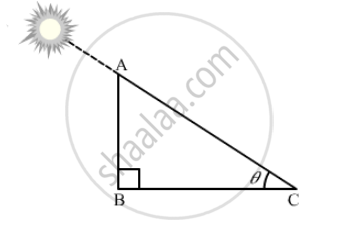Advertisement Remove all ads

# The length of shadow of a tower on the plane ground is √ 3 times the height of the tower. The angle of elevation of sun is - Mathematics

MCQ

The length of shadow of a tower on the plane ground is $\sqrt{3}$  times the height of the tower. The angle of elevation of sun is

#### Options

• 45°

•  30°

• 60°

• 90°

Advertisement Remove all ads

#### SolutionLet the angle of elevation of the sun be θ.

Suppose AB is the height of the tower and BC is the length of its shadow.

It is given that, BC = $\sqrt{3}$AB

In right ∆ABC,

$\tan\theta = \frac{AB}{BC}$

$\Rightarrow \tan\theta = \frac{AB}{\sqrt{3}AB} = \frac{1}{\sqrt{3}}$

$\Rightarrow \tan\theta = \tan30°$

$\Rightarrow \theta = 30°$

Thus, the angle of elevation of the sun is 30º.

Is there an error in this question or solution?
Advertisement Remove all ads

#### APPEARS IN

RD Sharma Class 10 Maths
Chapter 12 Trigonometry
Q 21 | Page 43
Advertisement Remove all ads

#### Video TutorialsVIEW ALL 

Advertisement Remove all ads
Share
Notifications

View all notifications

Forgot password?
Course